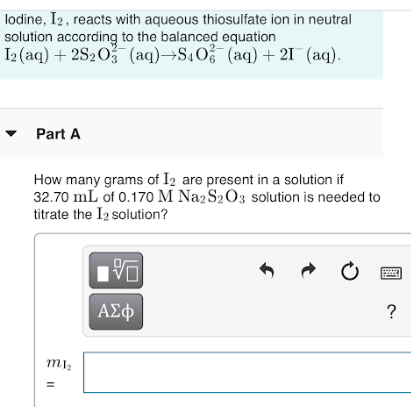# Iodine, I2, reacts with aqueous thiosulfate ion in neutral solution according to the balanced equation. I2(aq) + 2S2O^3 (aq) → S4O6 (aq) + 2I^- (aq). How many grams of I2 are present in a solution if 32.70 mL of 0.170 M Na2S2O3 solution is needed to titrate the I2 solution?